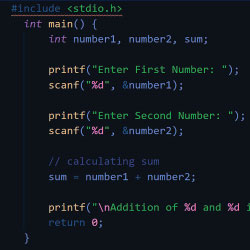# What are the conditional operators in C?

Updated By: Alka Patel

A

Atul Verma

08 November 2021

### Conditional Operator in C Programming

In C programming conditional operator is also called a ternary operator. Technically ternary operator is the short form of an if-else statement. In the if-else statement, if the condition is true then the scope of if condition will execute, and if the condition is false then the scope of else will execute.

Same as the if-else statement in ternary operator if the condition is true then expression1 will execute and if the condition is false then expression2 will execute.

#### Let's see the Syntax of Conditional Operator in C

condition ? expression 1 : expression 2

In the conditional operator, there are three operands, hence it is also called a ternary operator.

Let's understand the ternary operator by taking an example

int a=5;

(a==5) ? printf("The number is 5") : printf("The number is not 5");

OUTPUT

The number is 5

In above example

condition is: (a==5)

expression 1 is: printf("The number is 5")

expression 2 is: printf("The number is not 5")

Extra Points

We can assign the result of the ternary operator to a variable like below example

int a=10

b = ((a==9)? (5) : (7));

printf("The value of b is: %d", b)

OUTPUT

The value of b is: 7

So you can use conditional operator in two ways i.e. running some block of codes based on some condition, and getting the value of complete ternary operator, we can store this value in a variable and then we can perform some other tasks based on that variable.

I hope now your all query regarding conditional operator will be resolved, still, if you are facing a problem in understanding conditional operator then write in the comment box I will try to answer it and if you have more details about ternary operator kindly share with us, we all would like to know your information about the conditional operatorTop 10 most handsome Chinese actors
20 November 2021 | 12:00 AM